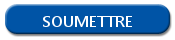# Logical and arithmetic operators in R

## Data processing, logical and arithmetic operator in Rs

The R language have several operators for data processing. Let's review these operators.

## Arithmetic operators in R

Find below the main arithmetic operators used for computer programming.

 Operator Function + Addition - Subtraction * Multiplication / division ^ exponent %% module %/% Euclidean division

## Logical operators in R

In the same way, the logical operators used in R have the same base than the other programming languages. For example, you can use these operators for your conditions.

 Operator Function < inferior > superior <= Inferior or equal >= Superior or equal == equal != different | or & and